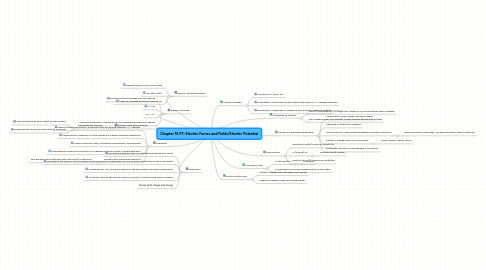# Chapter 16/17: Electric Forces and Fields/Electric Potential

Get Started. It's FreeChapter 16/17: Electric Forces and Fields/Electric Potential## 3. Means of Charging/Discharging

### 3.3. Semiconductor: intermediate between insulator/conductor

3.3.1. Photoconductor: uses light--no light=insulator, light=conductor

### 3.4. Insulator: charge does not move easily

3.4.1. Glass, plastic, rubber, wood

4.1.1. k=8.99e9

## 5. Calculations

### 5.2. F=(k*q*Q)/r2

5.2.1. Q=single point charge

## 9. Electric Field and Potential:

### 9.1. Equipotential Surface

9.1.1. Same potential at every point on the surface

9.1.2. perpendicular to electric field lines at all points

## 10. Capacitor

### 10.5. Parallel Plate Capacitor(simplest)

10.5.1. two parallel plates with the same area set at a distance